News/Noticias‎ > ‎

### 42 - Hitchhiker's Guide to the Galaxy by Douglas Adams

posted May 26, 2013, 6:16 PM by Jose L. Mendoza-Cortes
In The Hitchhiker's Guide to the Galaxy by Douglas Adams, the number 42 is the "Answer to the Ultimate Question of Life, the Universe, and Everything". But he didn't say what the question was!

Let me reveal that now.

If you try to get several regular polygons to meet snugly at a point in the plane, what's the most sides any of the polygons can have? The answer is 42.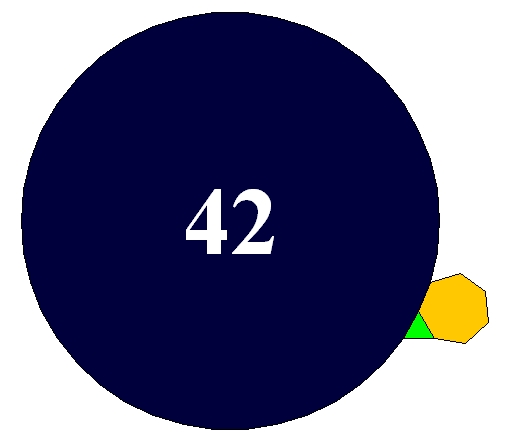The picture shows an equilateral triangle, a regular heptagon and a regular 42-gon meeting snugly at a point. The reason this works is that
13+17+142=12There are actually 17 solutions of
1p+1q+1r=12with p≤q≤r, and each of them gives a way for three regular polygons to snugly meet at a point. But this particular solution features the biggest number of all!

But why is this so important? Well, it turns out that if you look for natural numbers a,b,c that make
1a+1b+1cas close to 1 as possible, while still less than 1, the very best you can do is 1/2+1/3+1/7. It comes within 1/42 of equalling 1, since
13+17+142=12

And why is this important? Well, suppose you're trying to make a doughnut with at least two holes that has the maximum number of symmetries. More precisely, suppose you're trying to make a Riemann surface with genus g≥2 that has the maximum number of conformal transformations. Then you need to find a highly symmetrical tiling of the hyperbolic plane by triangles whose interior angles are π/a,π/b and π/c, and you need
1a+1b+1c<1for these triangles to fit on the hyperbolic plane.

For example, if you take a=2,b=3,c=7 you get this tiling: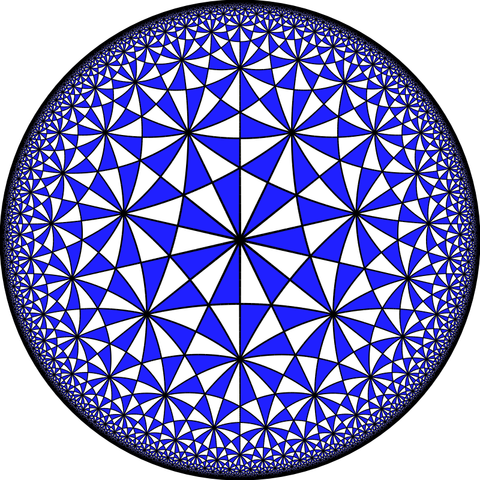A clever trick then lets you curl up the hyperbolic plane and get a Riemann surface with at most
2(g−1)1−1a−1b−1csymmetries. So, you want to make 1−1a−1b−1c as small as possible! And thanks to what I said, the best you can do is
1−12−13−17=142So, your surface can have at most
84(g−1)symmetries. This is called Hurwitz's automorphism theorem. The number 84 looks mysterious when you first see it — but it's there because it's twice 42.

But why is this stuff the answer to the ultimate question of life, the universe, and everything? I'm not sure, but I have a crazy theory. Maybe all matter and forces are made of tiny little strings! As they move around, they trace out Riemann surfaces in spacetime. And when these surfaces are as symmetrical as possible, reaching the limit set by Hurwitz's automorphism theorem, the size of their symmetry group is a multiple of 42, thanks to the math I just described.
PuzzlesI mentioned two facts that are actually pretty fun and easy to check. So, try these puzzles:

Puzzle 1. Consider solutions of
1p+1q+1r=12with positive integers p≤q≤r, and show that the largest possible value of r is 42.

Puzzle 2. Consider solutions of
1a+1b+1c<1with positive integers a,b,c, and show that the largest possible value of 1a+1b+1c is 1−142.

Appendix: more details and referencesI said the maximum number of conformal transformations of a Riemann surface with g≥2 holes is 84(g−1). However, we can't always get that many. For example, we can't find a Riemann surface with 2 holes that has 84 symmetries of this type.

The first time we reach the maximum is when our surface has 3 holes. Starting in 1878, Felix Klein studied the most symmetrical Riemann of this sort, and he showed it has 84×2=168 symmetries. It's a really amazing thing, called Klein's quartic curve. Here's a picture, made by Greg Egan:If you carefully count the triangles in the picture above, you'll get 56. However, these triangles are equilateral, or at least they would be if we could embed Klein's quartic curve in 3d space without distorting it. If we drew all the smaller triangles whose interior angles are π/2,π/3 and π/7, each equilateral triangle would get subdivided into 6 smaller ones, and there would be a total of 6×56=336 of these. But
336=8×42Half of these smaller triangles are 'left-handed' and half are 'right-handed'. There's a conformal transformation sending a chosen triangle to any other triangle of the same handedness, for a total of
168=4×42transformations.

The next case where we get the maximum number of symmetries is the 7-holed torus. This can be given a complex structure whose that admits 504=84×6 conformal transformations. This is called the Fricke–Macbeath curve, discovered by Fricke in 1899.

The next case is the 14-holed torus, which can be given three different complex structures with 1092 = 84 × 13 symmetries. These are called the first Hurwitz triplet.

This is the start of a complicated and interesting subject. For more details, see my page on Klein's quartic curve, and especially the section on incredibly symmetrical surfaces.

Sums of reciprocals of natural numbers are called 'Egyptian fractions', and they have deep connections to geometry; for more on this see my article on Archimedean tilings and Egyptian fractions.

The picture of a triangle, heptagon and 42-gon was made by Tyler, and you can see all 17 ways to get 3 regular polygons to meet snugly at a vertex on Wikipedia. Of these, only 11 can occur in a uniform tiling of the plane. The triangle, heptagon and 42-gon do not tile the plane, but you can see some charming attempts to do something with them on Kevin Jardine's website Imperfect Congruence: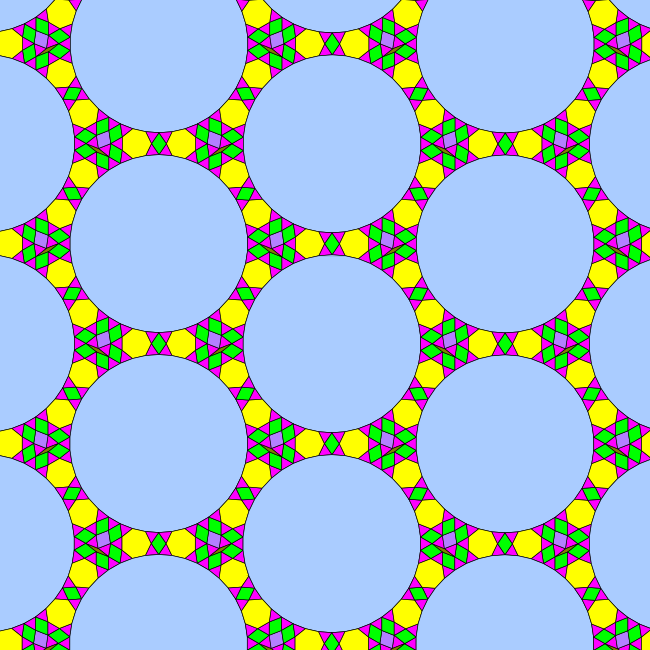Puzzle 1. Consider solutions of
1p+1q+1r=12with positive integers p≤q≤r, and show that the largest possible value of r is 42.

Answer. We will systematically go through positive integers p≤q with 1p+1q<12, working our way up from small integers to big ones, so that 1p+1q decreases, forcing 1r to get bigger and thus forcing r to go down.
p=1: here 1p is already bigger than 12, so this can't work.
p=2: here 1p is already equal to 12, so this can't work.
p=3: now there's hope.

q=3: here 1p+1q=13+13 is already bigger than 12, so this can't work.
q=4: 13+14 is still bigger than 12.
q=5: 13+15 is still bigger than 12.
q=6: 13+16 is equal to 12, so this still can't work.
q=7: 13+17 is smaller than 12, so finally there's hope, and
13+17+142=12so this gives a solution (p,q,r)=(2,7,42) with 42 as its biggest number, and this is the winner. We could stop here, but just to be careful...
q=8: 13+18 is even smaller, and
13+18+124=12so this gives a solution (p,q,r)=(2,8,24) with 24 as its biggest number. This turns out to be the runner-up in the contest to make r as big as possible.
q=9: 13+19 is even smaller, and
13+19+118=12so this gives a solution (p,q,r)=(2,9,18) with 18 as its biggest number. Let us quit marching down this path, and see what happens if we try the next choice of p.

p=4: this also allows for solutions.

q=4: 14+14 is equal to 12, so this can't work.
q=5: 14+15 is smaller than 12, and
14+15+120=12so this gives a solution (p,q,r)=(4,5,20) with 20 as its biggest number. This turns out to be the third best solution.And so on: it's all downhill from here. The solutions we've found gives ways to make 3 regular polygons meet snugly at a vertex:
13+17+142=12. This one gives the winner for the polygon with the most sides: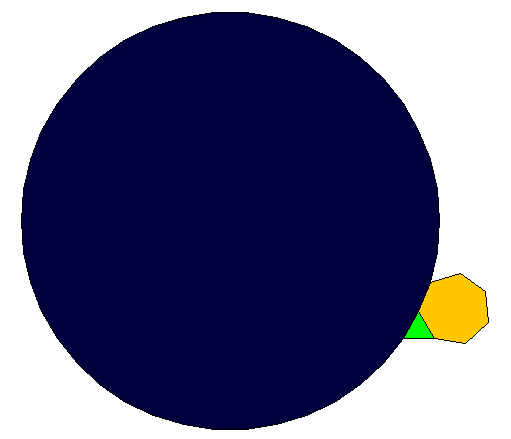13+18+124=12. This is the second best: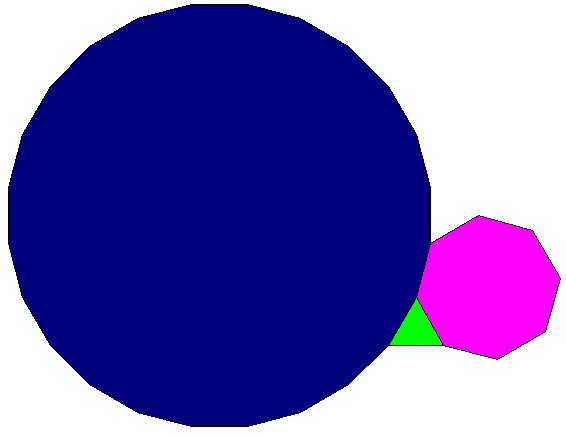14+15+120=12. This is the third best: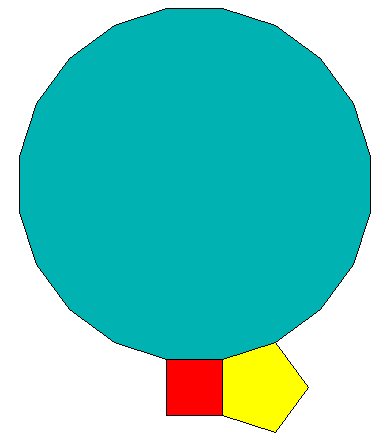13+19+118=12. This is the fourth best: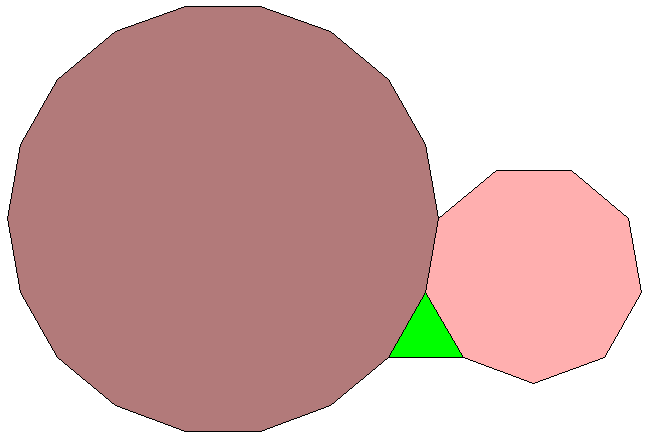If you want a harder puzzle, you can march on and figure out all the solutions of
1p+1q+1r=12

Puzzle 2. Consider solutions of
1a+1b+1c<1with positive integers a,b,c, and show that the largest possible value of 1a+1b+1c is 1−142.

Answer. The same sort of approach works here. We can assume a≤b≤c.
a=1: here 1a is already 1, so this can't work.
a=2: now there's hope.

b=2: here 1a+1b=12+12 is already 1, so this can't work.
b=3: now 1a+1b=12+13=56, so there's hope. We have 12+13+16=1, so the closest we can get to 1 without equalling 1 is
12+13+17=1−142This solution, (a,b,c)=(2,3,7), turns out to be the winner. We also have solutions like (a,b,c)=(2,3,8) and so on, which don't come as close to 1:
12+13+18=1−124But just to be careful...
b=4: now 1a+1b=12+14=34, so there's hope. We have 12+14+14=1, so the closest we can get to 1 without equalling 1 is
12+14+15=1−120So, this solution (a,b,c)=(2,4,5) doesn't beat the winner! And neither does anything else.Each solution of
1a+1b+1c<1with positive integers a,b,c gives a tiling of hyperbolic space by triangles whose interior angles are π/a,π/b and π/c. There are infinitely many solutions, but we have found the three 'flattest' ones, meaning that the triangles are closest to Euclidean: their interior angles add up to almost π:
(2,3,7): the sum of interior angles is π−π/42:(2,3,8): the sum of interior angles is π−π/24: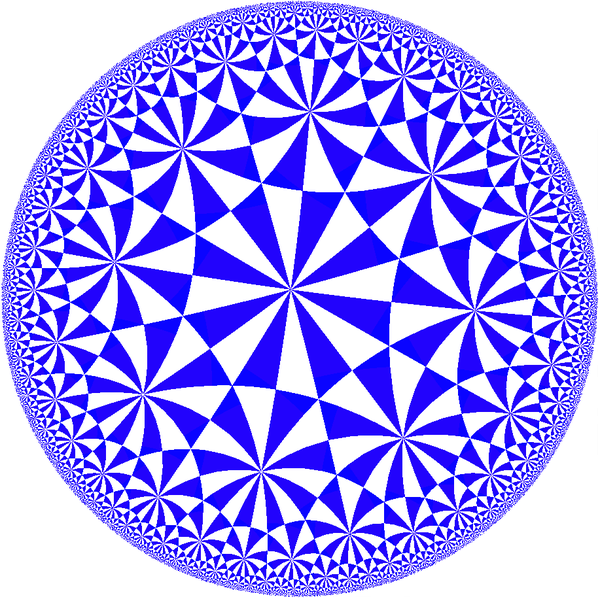(2,4,5): the sum of interior angles is π−π/20:There is a lot one can do with these tilings, starting here.

"Good Morning," said Deep Thought at last.
"Er...good morning, O Deep Thought" said Loonquawl nervously, "do you have...er, that is..."
"An Answer for you?" interrupted Deep Thought majestically. "Yes, I have."
The two men shivered with expectancy. Their waiting had not been in vain.
"There really is one?" breathed Phouchg.
"There really is one," confirmed Deep Thought.
"To Everything? To the great Question of Life, the Universe and everything?"
"Yes."
Both of the men had been trained for this moment, their lives had been a preparation for it, they had been selected at birth as those who would witness the answer, but even so they found themselves gasping and squirming like excited children.
"And you're ready to give it to us?" urged Loonsuawl.
"I am."
"Now?"
"Now," said Deep Thought.
They both licked their dry lips.
"Though I don't think," added Deep Thought. "that you're going to like it."
"Doesn't matter!" said Phouchg. "We must know it! Now!"
"Now?" inquired Deep Thought.
"Yes! Now..."
"All right," said the computer, and settled into silence again. The two men fidgeted. The tension was unbearable.
"You're really not going to like it," observed Deep Thought.
"Tell us!"
"All right," said Deep Thought. "The Answer to the Great Question..."
"Yes...!"
"Of Life, the Universe and Everything..." said Deep Thought.
"Yes...!"
"Is..." said Deep Thought, and paused.
"Yes...!"
"Is..."
"Yes...!!!...?"
"Forty-two," said Deep Thought, with infinite majesty and calm. - Douglas Adams, The Hitchhiker's Guide to the Galaxy